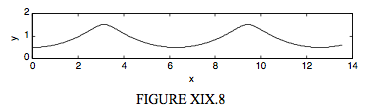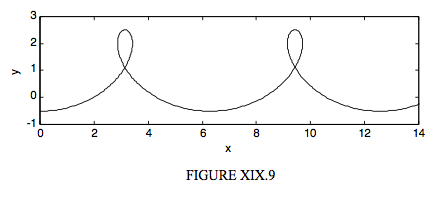$$\require{cancel}$$

# 19.8: Contracted and Extended Cycloids

•• Contributed by Jeremy Tatum
• Emeritus Professor (Physics & Astronomy) at University of Victoria

As in Section 19.1, we consider a circle of radius a rolling to the right on the line $$y = 2a$$. The point P is initially below the centre of the circle, but, instead of being on the rim of the circle, its distance from the centre of the circle is $$r$$. If $$r < a$$, the path described by P will be a contracted cycloid; if $$r > a$$, the path is an extended cycloid. (I think there’s a case for using this nomenclature the other way round, but most authors seem to use “contracted” for $$r < a$$ and “extended” for$$r > a$$.) It should not take long to be convinced, by arguments similar to those in Section 19.1, that the parametric equations to a contracted or extended cycloid are

$x = 2 a \theta + r \sin 2 \theta \label{19.8.1}\tag{19.8.1}$

and

$y = a - r \cos 2 \theta \label{19.8.2}\tag{19.8.2}$

These are illustrated in Figures XIX.8 and XIX.9 for a contracted cycloid with $$r = 0.5a$$ and an extended cycloid with $$r = 1.5a$$.